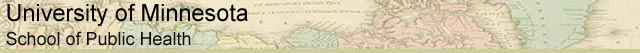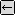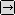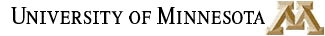### MATH REVIEW: USEFUL MATH FOR EVERYONE

SECTION 4.3. WHAT IS A LOGARITHM?back to Logarithms, Page 2

### Logarithmic Rules

Just as exponents have some basic rules that make them easier to manipulate (see Section 3: Exponents), so do logarithms. These rules apply to all logarithms, including base 10 logarithms and natural logarithms. For simplicity's sake, base ten logs are used in most of these rules:

1.   br = a is the equivalent to logb a=r (This is the definition of a logarithm.)

2.   log 0 is undefined.

3.   log 1 = 0

4.   log (P*Q) = log P + log Q

5.   log (P/Q) = log P - log Q

6.   log (Pt) = t *log P

7.   10(log a) = a (in the case of natural logarithms, e(ln a) = a)

8.   log (10r) = r (in the case of natural logarithms, ln er = r)

9.   log (1/a) = -log a

Let's take a closer look at each of these rules:

1. br = a is the equivalent of logb a=r. We've already looked at how this works, but here's another example:

log 14 ≈ 1.146

is the equivalent of

10 1.146 ≈ 14

2. log 0 is undefined. It's not a real number, because you can never get zero by raising anything to the power of anything else. You can never reach zero, you can only approach it using an infinitely large and negative power.

3. log 1 = 0 means that the logarithm of 1 is always zero, no matter what the base of the logarithm is. This is because any number raised to 0 equals 1. Therefore, ln 1 = 0 also.

All the rest of the logarithmic rules are useful for solving complex equations, or equations with unknowns.to Logarithms, Page 4